International
Tables for
Crystallography
Volume D
Physical properties of crystals
Edited by A. Authier

International Tables for Crystallography (2013). Vol. D, ch. 1.11, pp. 278-279

## Section 1.11.6.5. Tensor atomic factors (magnetic case)

V. E. Dmitrienko,a* A. Kirfelb and E. N. Ovchinnikovac

aA. V. Shubnikov Institute of Crystallography, Leninsky pr. 59, Moscow 119333, Russia,bSteinmann Institut der Universität Bonn, Poppelsdorfer Schloss, Bonn, D-53115, Germany, and cFaculty of Physics, M. V. Lomonosov Moscow State University, Leninskie Gory, Moscow 119991, Russia
Correspondence e-mail:  dmitrien@crys.ras.ru

#### 1.11.6.5. Tensor atomic factors (magnetic case)

| top | pdf |

Magnetic crystals possess different densities of states with opposite spin directions. During a multipole transition from the ground state to an excited state (or the reverse), the projection of an electron spin does not change, but the projection of the orbital moment varies. The consideration of all possible transitions allows for the formulation of the sum rules (Carra et al., 1993; Strange, 1994) that are widely used in X-ray magnetic circular dichroism (XMCD). When measuring the differences of the absorption coefficients at theabsorption edges of transition elements or at the M edges of rare-earth elements (Erskine & Stern, 1975; Schütz et al., 1987; Chen et al., 1990), these rules allow separation of the spin and orbital contributions to the XMCD signal, and hence the study of the spin and orbital moments characterizing the ground state. In magnetic crystals, the tensors change their sign with time reversal becauseif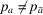and/or(Zeeman splitting in a magnetic field). That the antisymmetric parts of the tensors differ from zero follows from equations (1.11.6.7), (1.11.6.10)and (1.11.6.15).

Time reversal also changes the incident and scattered vectors corresponding to permutation of the Cartesian tensor indices. For dipole–dipole resonant events, the symmetric part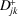does not vary with exchange of indices, hence it is time- and parity-even. The antisymmetric part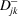changes its sign upon permutation of the indices, so it is parity-even and time-odd, being associated with a magnetic moment (1.11.6.41). This part of the tensor is responsible for the existence of X-ray magnetic circular dichroism (XMCD) and the appearance of the magnetic satellites in various kinds of magnetic structures.

If the rotation symmetry of a second-rank tensor is completely described by rotation about the magnetic moment m, then the antisymmetric second-rank tensorcan be represented as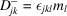, where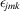is an antisymmetric third-rank unit tensor andare the coordinates of the magnetic moment of the resonant atom. So, the scattering amplitude for the dipole–dipoletransition can be given as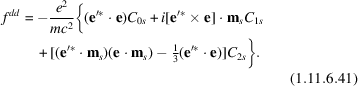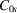,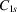and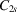are energy-dependent coefficients referring to the sth atom in the unit cell and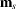is a unit vector along the magnetic moment. The third term in (1.11.6.41)is time non-reversal, and it is responsible for the magnetic linear dichroism (XMLD). This kind of X-ray dichroism is also influenced by the crystal field (Thole et al., 1986; van der Laan et al., 1986).

The coefficients,andinvolved in (1.11.6.41)may be represented in terms of spherical harmonics. Using the relations (Berestetskii et al., 1982; Hannon et al., 1988)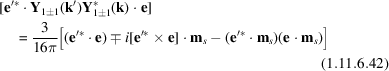and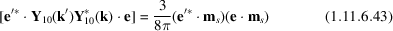for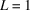,and,, respectively, one obtains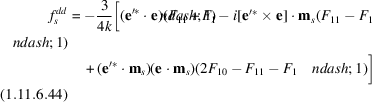with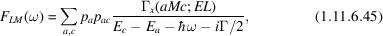where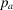is the probability of the initial state,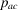is that for the transition from stateto a final state, and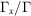is the ratio of the partial line width of the excited state due to a pure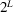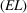radiative decay and the width due to all processes, both radiative and non-radiative (for example, the Auger decay).

Magnetic ordering is frequently accompanied by a local anisotropy in the crystal. In this case, both kinds of local anisotropies exist simultaneously and must be taken into account in, for example, XMLD (van der Laan et al., 1986) and XMχD (Goulon et al., 2002). In resonant X-ray scattering experiments, simultaneous existence of forbidden reflections provided by spin and orbital ordering (Murakami et al., 1998) as well as magnetic and crystal anisotropy (Ji et al., 2003; Paolasini et al., 2002, 1999) have been observed. The explicit Cartesian form of the tensor atomic factor in the presence of both a magnetic moment and crystal anisotropy has been proposed by Blume (1994). When the symmetry of the atomic site is high enough, i.e. the atom lies on an n-order axis (), then the tensors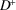and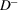can be represented asand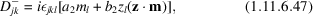whereanddepend on the energy, andis a unit vector along the symmetry axis under consideration. One can see that the atomic tensor factor is given by a sum of three terms: the first is due to the symmetry of the local crystal anisotropy, the second describes pure magnetic scattering, and the last (combined') term is induced by interference between magnetic and non-magnetic resonant scattering. This issue was first discussed by Blume (1994) and later in more detail by Ovchinnikova & Dmitrienko (1997, 2000). All the terms can give rise to forbidden reflections, i.e. sets of pure resonant forbidden magnetic and non-magnetic reflections can be observed for the same crystal, see Ji et al. (2003) and Paolasini et al. (2002, 1999). Only reflections caused by the combined' term (Ovchinnikova & Dmitrienko, 1997) have not been observed yet.

Neglecting the crystal field, an explicit form of the fourth-rank tensors describing the quadrupole–quadrupole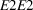events in magnetic structures was proposed by Hannon et al. (1988) and Blume (1994):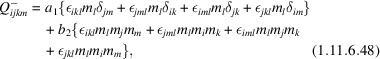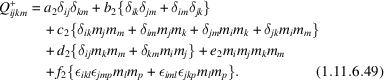Then, being convoluted with polarization vectors, the scattering amplitude of the quadrupole transition () can be written as a sum of 13 terms belonging to five orders of magnetic moments (Hannon et al., 1988; Blume, 1994). The final expression that gives the quadrupole contribution to the magnetic scattering amplitude in terms of individual spin components is rather complicated and can be found, for example, in Hill & McMorrow (1996). In the presence of both a magnetic moment and local crystal anisotropy, the fourth-rank tensor describingevents depends on both kinds of anisotropy and can include the `combined' part in explicit form, as found by Ovchinnikova & Dmitrienko (2000).

### References

Berestetskii, V. B., Lifshitz, E. M. & Pitaevskii, L. P. (1982). Quantum Electrodynamics. Oxford: Pergamon Press.Google Scholar
Blume, M. (1994). Resonant X-ray diffraction and polarization analysis at the iron K-edge. In Resonant Anomalous X-ray Scattering. Theory and Applications, edited by G. Materlik, C. J. Sparks & K. Fischer, pp. 91–97. Amsterdam: North-Holland.Google Scholar
Carra, P., Thole, B. T., Altarelli, M. & Wang, X. (1993). X-ray circular dichroism and local magnetic fields. Phys. Rev. Lett. 70, 694–697.Google Scholar
Chen, C. T., Sette, F., Ma, Y. & Modesti, S. (1990). Soft X-ray magnetic circular dichroism at the L2,3 edges of nickel. Phys. Rev. B, 42, 7363–7265.Google Scholar
Erskine, J. L. & Stern, E. A. (1975). Calculation of the M23 magneto-optical absorption spectrum of ferromagnetic nickel. Phys. Rev. B, 12, 5016–5024.Google Scholar
Goulon, J., Rogalev, A., Wilhelm, F., Goulon-Ginet, C. & Carra, P. (2002). X-ray magnetochiral dichroism: a new spectroscopic probe of parity nonconserving magnetic solids. Phys. Rev. Lett. 88, 237401.Google Scholar
Hannon, J. P., Trammell, G. T., Blume, M. & Gibbs, D. (1988). X-ray resonance exchange scattering. Phys. Rev. Lett. 61, 1245–1248.Google Scholar
Hill, J. P. & McMorrow, D. F. (1996). Resonant exchange scattering: polarization dependence and correlation function. Acta Cryst. A52, 236–244.Google Scholar
Ji, S., Song, C., Koo, J., Lee, K.-B., Park, Y. J., Kim, J. Y., Park, J.-H., Shin, H. J., Rhyee, J. S., Oh, B. H. & Cho, B. K. (2003). Interference of magnetic and ATS reflections in resonant X-ray scattering of GdB4. Phys. Rev. Lett. 91, 257205.Google Scholar
Laan, G. van der, Thole, B. T., Sawatzky, G., Goedkoop, J. B., Fuggle, J. C., Esteva, J.-M., Karnatak, R., Remeika, J. P. & Dabkowska, H. A. (1986). Experimental proof of magnetic X-ray dichroism. Phys. Rev. B, 34, 6529–6531.Google Scholar
Murakami, Y., Kawada, H., Kawata, H., Tanaka, M., Arima, T., Moritomo, Y. & Tokura, Y. (1998). Direct observation of charge and orbital ordering in La0.5Sr1.5MnO4. Phys. Rev. Lett. 80, 1932–1935.Google Scholar
Ovchinnikova, E. N. & Dmitrienko, V. E. (1997). Combined effects of magnetic structure and local crystal fields in anisotropic X-ray anomalous scattering. Acta Cryst. A53, 388–395.Google Scholar
Ovchinnikova, E. N. & Dmitrienko, V. E. (2000). Resonant X-ray scattering in the presence of several anisotropic factors. Acta Cryst. A56, 2–10.Google Scholar
Paolasini, L., Caciuffo, R., Sollier, A., Ghigna, P. & Altarelli, M. (2002). Coupling between spin and orbital degrees of freedom in KCuF3. Phys. Rev. Lett. 88, 106403.Google Scholar
Paolasini, L., Vettier, C., de Bergevin, F., Yakhou, F., Mannix, D., Stunault, A., Neubeck, W., Altarelli, M., Fabrizio, M., Metcalf, P. & Honig, J. (1999). Orbital occupancy order in V2O3: resonant X-ray scattering results. Phys. Rev. Lett. 82, 4718–4722.Google Scholar
Schütz, G., Wagner, W., Wilhelm, W., Kenle, P., Zeller, R., Frahm, R. & Materlik, G. (1987). Absorption of circularly polarized X-rays in iron. Phys. Rev. Lett. 58, 737–740.Google Scholar
Strange, P. (1994). Magnetic absorption dichroism and sum rules in itinerant magnets. J. Phys. Condens. Matter, 6, L491–L495.Google Scholar
Thole, B. T., van der Laan, G. & Sawatzky, G. (1986). Strong magnetic dichroism predicted in the M4,5 X-ray absorption spectra of magnetic rare-earth materials. Phys. Rev. Lett. 55, 2086–2088.Google Scholar